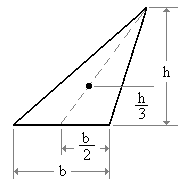# Splitting a convex set through its center

Three days ago I raised the question How unevenly can you split a convex set through its center? Scott had asked in the comments what kind of sets split most unevenly through their centers. I didn’t have an answer at the time, but now I have something.

Start with a triangle. Draw a line from the middle of the base up to the top. The center of mass is located 1/3 of the way up that line.Now draw a horizontal line through the center of mass. The triangle above this line is similar to the entire triangle, but will have 2/3 of the original height. By similarity, it also has 2/3 of the base, and so it has 4/9 of the area of the entire triangle. The portion below the center of mass has 5/9 of the area.

Now let’s go up a dimension and imagine a tetrahedron sitting on a table. Draw a line from the center of the base up to the top. The center of mass for the tetrahedron will be located along that line, 1/4 of the way up. Pass a plane through the center of mass parallel to the table. This forms a new tetrahedron above the cutting plane, similar to the original tetrahedron. The new tetrahedron has 3/4 of the height, and by similarity, it has (3/4)3 = 27/64 of the original volume. Of course the solid below the cutting plane then has 37/64 of the original volume.

Now consider the general case. The center of mass of an n-simplex is located 1/(n+1) of the way up along a line running from the center of the base to the top. Cut the n-simplex through the center of mass with a hyperplane parallel to the base. The n-simplex above the hyperplane has n/(n+1) of the original height and (n/(n+1))n of the original volume. As n goes to infinity, this expression converges to 1/e.

In summary, we’ve shown that a convex set in Rn can have at least 1 – (n/(n+1))n of its volume on one side of a hyperplane through the center of mass by constructing such a set, namely any n-simplex. And as n goes to infinity, this volume approaches 1 – 1/e. We have not shown that an n-simplex is the worst case in each dimension n, though I suspect this is true.  If you take a ball in Rn, any plane through the center divides the ball exactly in half. In some sense, an n-simplex is as far as a convex set can get from a ball. It’s the least-round convex shape.

Update: Here’s a paper on the topic. B. Grunbaum, “Partitions of mass-distributions and convex bodies by hyperplanes,” Paciﬁc Journal of Mathematics. 10, 1257–1261, 1960.

## One thought on “Splitting a convex set through its center”

1. Thanks for the further discussion, John. It is now clear where the 1-1/e is coming from. Not only a tetrahedron, but a cone with any shaped-base will give the same result for dimension 3. Likewise for higher dimensions, I’m sure. The result as you now present it seems quite reasonable, but I’m still not sure how to prove it.